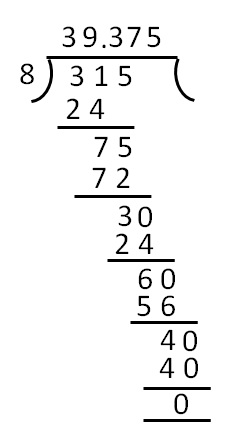Compound Interest for fraction years

Chapter 8 Class 8 Comparing Quantities
Concept wise

Let's do this by taking an example

## For Rs 10,000 at 10% p.a. What would be the compound interest for 2 1/2 years?

Given,

Principal = Rs 10,000

R = 10% p.a

Time = 2 1/2 years

Since time is in fraction,

we use the formula

Compound interest for 2 1/2 years

= Compound interest for 2 years

+ SI for next 1/2 years

### Compound interest for 2 years

Amount = P (1 + R/100) t

= 10,000 (1 + 10/100) 2

= 10,000 (1 + 1/10) 2

= 10,000 ((10 + 1)/10) 2

= 10,000 (11/10) 2

= 10,000 × (121/100)

= 100 × 121

= Rs 12,100

Now,

Amount = Principal + Interest

12,100 = 10,000 + Interest

12,100 − 10,000 = Interest

2100 = Interest

Interest = Rs 2100

### Simple interest for 1/2 years

Principal will be the amount after 2 years

P = Rs 12100

R = 5% p.a

T = 1/2 years

SI = PRT/100

= (12100 × 5 × 1/2)/100

= (12100 × 5)/(100 × 2)

= (121 × 5)/2

= 605/2

= Rs 302.5

Simple interest for 1/2 years = Rs 302.5

Now,

Compound interest for 2 1/2 years

= Compound interest for 2 years  + SI for next 1/2 years

= 2,100 + 302.5

= Rs 2402.5

∴ Compound interest after 2 1/2 years = Rs 2402.5

## Suppose I have Rs 1000 and I put it in a bank on compound interest. What would be the amount I have after 1 3/4 years, it interest rate is 5% pa.?

Given,

Principal = Rs 1000

Rate = 5% p.a

Time = 1 3/4 years

Since time is in fraction

We use the formula

Compound interest for 1 3/4 years

= Compound interest for 1 years  + SI for next 3/4 years

## Compound interest for 1 years

Amount = P (1 + R/100) T

= 1000 (1 + 5/100) 1

= 1000 (1 + 1/20)

= 1000 ((20 + 1)/20)

= 1000 (21/20)

= 100 × 21/2

= 50 × 21

= Rs 1050

Now,

Amount = Principal + Interest

1050 = 1000 + Interest

1050 − 1000 = Interest

50 = Interest

Interest = Rs 50

### Simple interest for 3 / 4 years

Principal will be the amount after 1 year

P = Rs 1050

R = 5% p.a

T = 3/4 years

S.I = PRT/100

= (1050 × 5 × 3/4)/100

= (1050 × 5 × 3)/(100 × 4)

= (105 × 5 × 3)/(10 × 4)

= (21 × 5 × 3)/(2 × 4)

= 315/8

= Rs 39.375Now,

Interest after 1 3/4 years = Compound interest for 1 year + Simple interest for next 3/4 year

= 50 + 39.375

= Rs 89.375

Get live Maths 1-on-1 Classs - Class 6 to 12